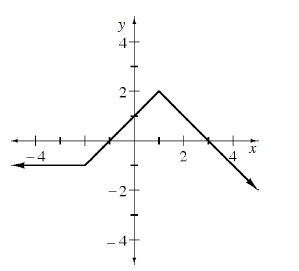### Home > CALC > Chapter 1 > Lesson 1.3.1 > Problem1-106

1-106.

Using the graph of $f(x)$ at right, sketch the following transformations: .

1. $-f(x)$

1. $f(x + 3)$

1. $f(x) - 2$

1. $|f(x)|$Use the eTool below to view the graphs of the transformations.
Click the link at right for the full version of the eTool: Calc 1-106 HW eTool